Next: Parameter Interpolation and Normalization Up: Implementation Previous: Implementation

## Lighting Model

Two kinds of surfaces can be distinguished according to the way they reflect light. On one hand, there are diffuse surfaces for which light is reflected in every direction (Figure 3(a)). On the other hand, there are specular surfaces for which light is reflected only in a small area around the mirror direction (Figure 3(b)).

 [Diffuse Reflection]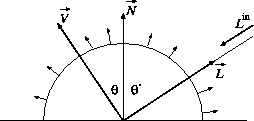[Specular Reflection]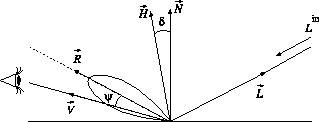There are many lighting models to describe this behavior. For simplicity we will use the Blinn-Phong  lighting model (Formula 1).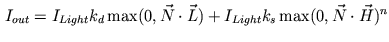(1)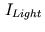is the color of the light,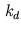is the diffuse color,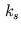is the specular color;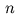is the specular exponent which defines the shininess of the surface.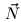is the normalized surface normal,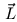is the normalized direction vector pointing to the light source. The half-angle vector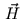is the half-way unit vector betweenand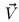defined in Formula 2.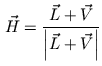(2)

Whereis the normalized vector to the viewer. It is important to max out negative dot product terms, as a negative dot product indicates that the point is in shadow and receives no light. The key aspects for successful per-pixel lighting are to provide the needed vector parameters (,and) for evaluating the lighting equation and to compute the per-pixel dot products. In the next Section we will see how to interpolate and normalize this vectors.Next: Parameter Interpolation and Normalization Up: Implementation Previous: Implementation
Gerald Schröcker 2002-03-21